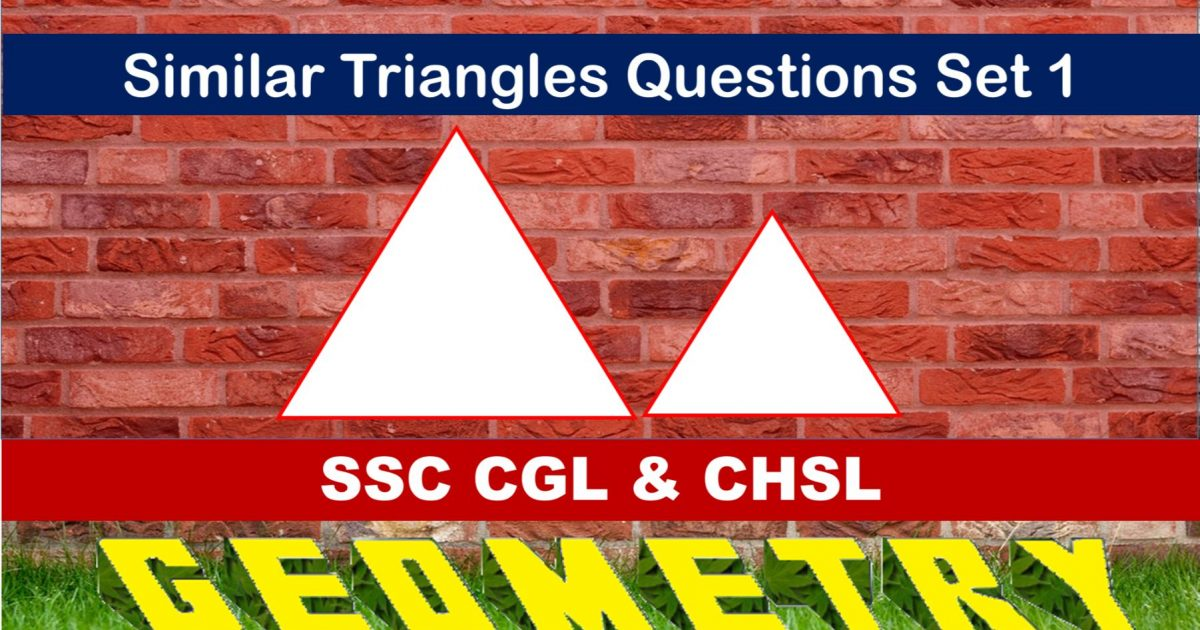Monday, September 27, 2021
Home > geometry > SSC CGL Geometry Similar Triangles Set 1

# SSC CGL Geometry Similar Triangles Set 1SSC CGL Geometry Similar Triangles Set 1 : Ques No 1

In triangle ABC, two points D and E are taken on the lines AB and BC respectively in such a way that AC is parallel to DE. The triangles ABC and DBE are

Options:

A. always similar

B. always congruent

C. similar  only if D lies outside the line segment AB

D. congruent only if D lies outside the line segment AB

Solution:

SSC CGL Geometry Similar Triangles Set 1 : Ques No 2

In a right triangle ABC, the angle A = 90 degree and AD is perpendicular to BC. If areas of the triangles ABC and ACD are 40 cm2 and 10 cm2 respectively and AC = 9 cm, then the length of BC is

Options:

A. 4 cm

B. 12 cm

C. 18 cm

D. 6 cm

Solution:

SSC CGL Geometry Similar Triangles Set 1 : Ques No 3

In a triangle ABC, D and E are two points on the sides AC and BC respectively, such that DE = 18 cm, CE = 5 cm and the angle DEC = 90 degree. If tan(∠ABC) = 3.6, then AD : CD is equal to

Options:

A. 2BC : CE

B. BC : 2CE

C. CE : 2BC

D. 2CE : BC

Solution:

SSC CGL Geometry Similar Triangles Set 1 : Ques No 4

In a triangle ABC, when the points D and E on sides AB and AC are joined, the line DE turns out to be parallel to BC and also bisects the triangle into two equal areas. The ratio of DB : AB is

Options:

A. 1 : 2

B. √2 : 1

C. (√2 – 1) : √2

D. 1 : √2

Solution:

SSC CGL Geometry Similar Triangles Set 1 : Ques No 5

Two similar triangles have the areas 96 cm2 and 150 cm2. If the largest side of the larger triangle is 20 cm, the largest side of the smaller triangle (in cm) is

Options:

A. 20

B. 15

C. 16

D. 18

Solution:

SSC CGL Geometry Similar Triangles Set 1 : Ques No 6

The perimeter of similar triangles ABC and PQR are 24 cm and 36 cm respectively. If AB = 10 cm, then PQ (in cm) is

Options:

A. 18

B. 12

C. 15

D. 16

Solution:SSC CGL Geometry Similar Triangles Set 1 : Ques No 7

In triangle ABC, the bisector of angle A is AP and it meets BC at P. If a line DE intersects AB, AP and AC at D, Q and E respectively and is perpendicular to AP, then which of the following is true?

Options:

A. AQ = QP

C. BP = PC

D. QP = EC

Solution:

SSC CGL Geometry Similar Triangles Set 1 : Ques No 8

In triangle ABC, DE || BC and AD : DB = 5 : 4. Then the ratio DE : BC is

Options:

A. 4 : 5

B. 9 : 5

C. 5 : 9

D. 4 : 9

Solution:

SSC CGL Geometry Similar Triangles Set 1 : Ques No 9

In a triangle ABC, BC = 12 cm. A line CD is drawn to intersect AB at D internally. If DB = 9 cm, CD = 6 cm and ∠BCD = ∠BAC, the ratio of the perimeter of ∆ADC to that of ∆BDC is

Options:

A. 5/9

B. 2/3

C. 7/9

D. 8/9

Solution:

SSC CGL Geometry Similar Triangles Set 1 : Ques No 10

In a triangle ABC, the line BB1 through B intersects side AC at B1. A line through A parallel to BB1, meets CB extended at A1 and another line through C parallel to BB1 meets AB extended at C1. Then, which of the following is true?

Options:

A. 1/AA1 – 1/CC1 = 2/BB1

B. 1/BB1 – 1/AA1 = 2/CC1

C. 1/CC1 + 1/AA1 = 1/BB1

D. 1/CC1 – 1/AA1 = 1/BB1

Solution:

SSC CGL Geometry Similar Triangles Set 1 : Ques No 11

AD is perpendicular to the internal bisector of ∠ABC of ∆ABC. DE is drawn through C and parallel to BC to meet AC at E. If the length of AC is 12 cm, then the length of AE (in cm) is

Options:

A. 3

B. 4

C. 6

D. 8